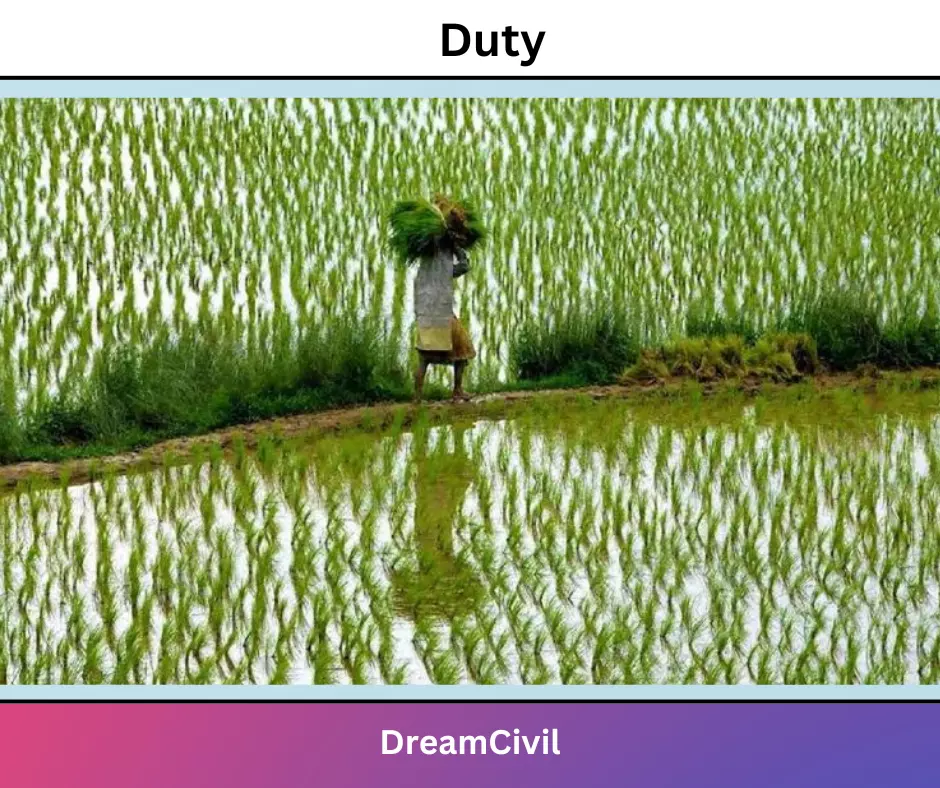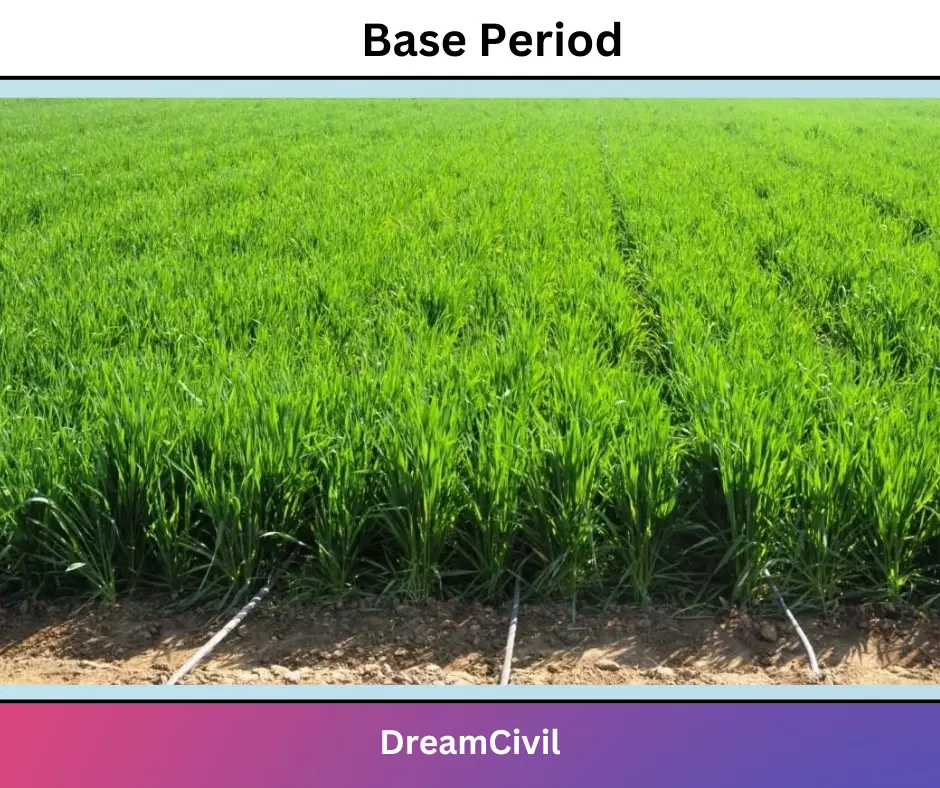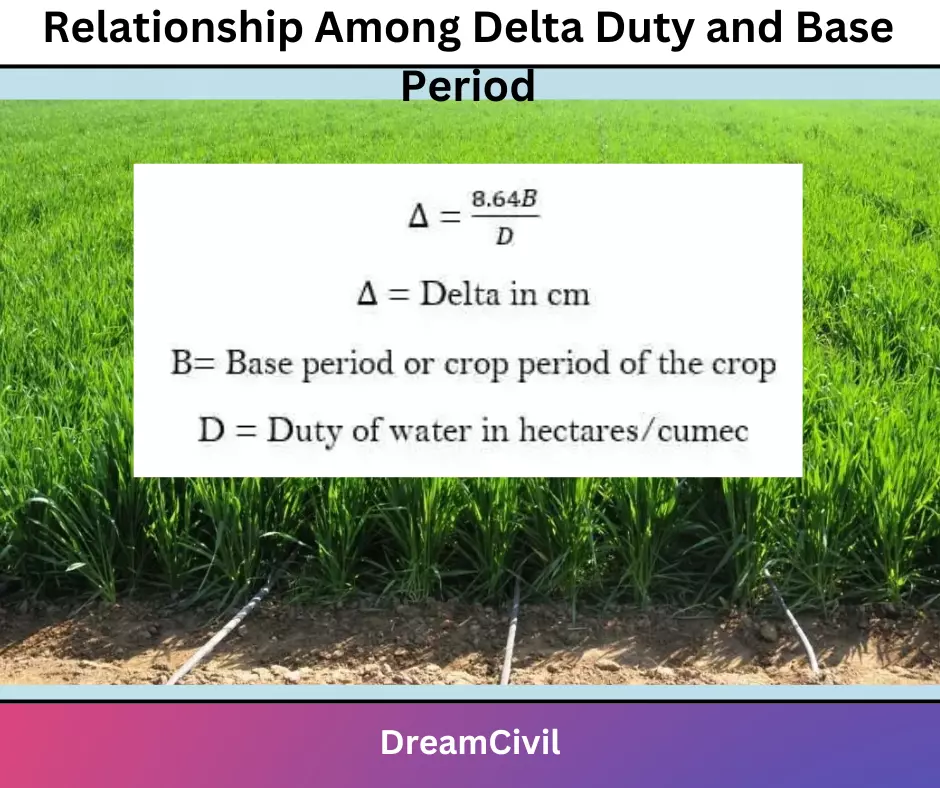Monday, September 25, 2023

# Delta Duty and Base Period Relationship : Derivation & Numerical

The relationship between Delta Duty and Base Period is given below with definitions.

### i. Duty

The duty of water is the relationship between the volume of water and the area of land it matures.

It may be defined as the area of land (in hectares) irrigated for complete growth of a given crop by continuously supplying 1 cumec of water during the entire base period of the crop.

Duty of water is generally expressed in hectare cumecs and is denoted by the letter `D `mathematically;

D=A/Q

Where,

D=Duty in hectare cumec

A=Area in hectares

Q=Discharge in m3/s

Duty can also be expressed as

D=8.44 B/Δ### ii. Delta

Each crop requires a certain amount of water after a fixed interval of time throughout its period of growth.

The water depth required varies between 5 to 10 cm depending upon the type of crops, climate & soil.

The interval between two consecutive watering is called the irrigation frequency or rotation period.

The depth of irrigation water required for a crop during its entire maturation period is called delta.

It is usually expressed In cms and is denoted by Δ.### iii. Base Period

The base period is the time between the first watering of a crop at the time of its sowing to its last watering before harvesting.

It is denoted by B.### iv. Relationship Among Delta Duty and Base Period ( Delta Duty Relationship)

Let us consider a crop having a base period of B days. Assume that 1 cumec of water is supplied to this crop for B days to provide delta Δ  m depth of water.

Now the volume of water supplied by unit discharge  to this crop during B days is given by:

V=Q (m³/s)*B (days)

V= 1*24*60*60*B m³   { B days = 24*60*60 Seconds  & Q = 1 m³/s  }

V=8.64*10000 B m³   …(1)

The volume of water applied to the field can also be calculated as:

V=Area of land irrigated by unit discharge * depth of water applied

V=D(ha)*Δ (m)

V=D *Δ*10000(m³)   ….(2)

From Eqn 1 & 2 , we get

8.864*10000*B= D*Δ*10000

D=(8.64*B)/Δ### v. Example

Calculate the delta based on the following data

Base peroid=110 days

Duty=1400ha/cumec

Delta=?

solution,

Δ =8.64*110/1400

Δ=0.68m

Δ =68cmHe is a founder and lead author of Dream Civil International and his civil engineering research articles has been taken as source by world's top news and educational sites like USA Today, Time, The richest, Wikipedia, etc.
Latest Articles

Related Articles